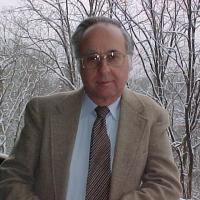Quantum Phase Space in Physics and Engineering
Speaker
Prof. Leon Cohen
City University of New York, USA
Email: leon.cohen@hunter.cuny.edu
Abstract

Quantum phase space originated in physics in 1932 and in engineering about 1942. The basic idea is to develop a joint distribution of position and momentum. We review the history of this development and its uses in quantum physics, engineering, statistical mechanics, noise theory, differential equations, classical dispersive wave propagation, and other related fields. We discuss their advantages and disadvantages, and the fundamental issues that arise in their use. In addition, we discuss how to transform differential equations into quantum phase space and the advantages of doing so. In Wigner's original 1932 paper, he transformed the Schrodinger equation into the equation that the associated Wigner distribution satisfies. We show how this can be generalized to ordinary and partial differential equations. We apply these methods to the propagation of pulses in dispersive media and derive new approximation methods. We also review the application of phase space distributions to non-stationary noise. Most of the development of noise theory has dealt with stationary noise. However, starting about 40 years ago, there has been a development that uses the ensemble average of the Wigner distribution to understand non-stationary noise. In signal analysis, the field is called time-frequency analysis. The fundamental aim is to understand how the frequency content of a signal is changing with time. We will explain how these methods are used to understand biomedical signals, music, radar and sonar signals, machine vibrations, animal sounds, and much more.